$$~$$

For anyone in social psychology using the Inclusion of the Other in the Self (IOS) Scale to measure self-other merging, you might have wished that you could know for sure how much self-other overlap there is between your participant and a target group or individual.

Indeed, the IOS response choices go from 1 to 7 only, and unfortunately these don’t match the percentage overlap from the circle images (i.e., 1/7 is not 14% overlap, and 7/7 is not 100% overlap). You can observe this on the original IOS scale below.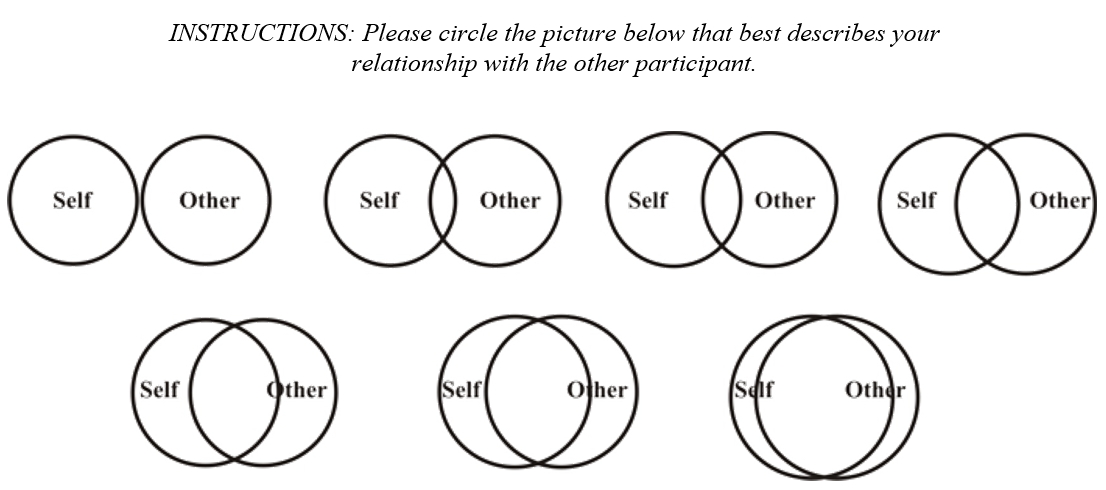So through trial and error (using the VennDiagram package), I was able to determine the approximate actual overlap from the pictures (below).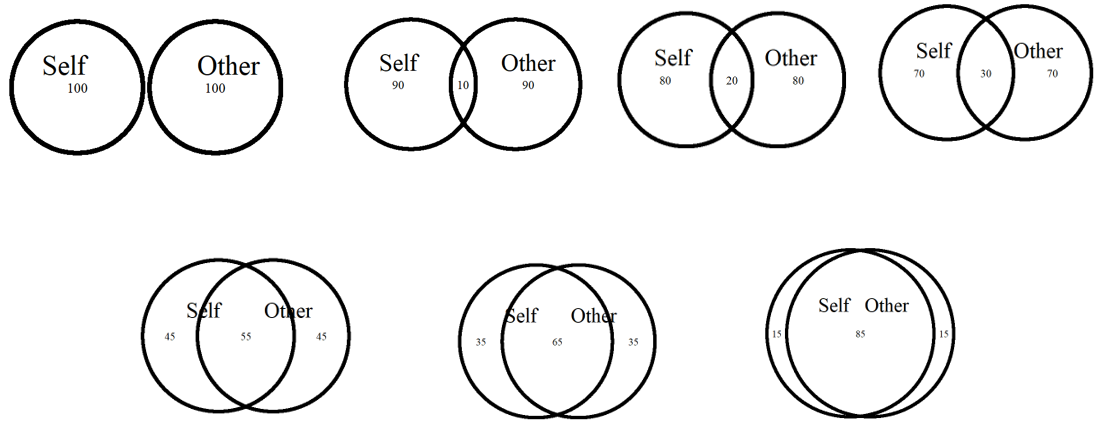I feel like the overlap is pretty close to the original scale. Based on this, I used the approx() function to interpolate any responses so that 1 = 0% (overlap), 2 = 10%, 3 = 20%, 4 = 30%, 5 = 55%, 6 = 65%, and 7 (the maximum) = 85%.

But that also means that a continuous response (for example based on a group average) can also be plotted accurately (e.g., a score of 6.84 would turn into 81.8 % overlap). Let’s see a few examples.

But first, let’s source the function from my github:

source("https://raw.githubusercontent.com/RemPsyc/niceplots/master/overlapCircleFunction.R")

### Score of 1 (0% overlap)

*Warning:* running the function below for the first time will install and load the following
Note: This will run many lines of code on your console and could take a few minutes.
overlapCircle(1)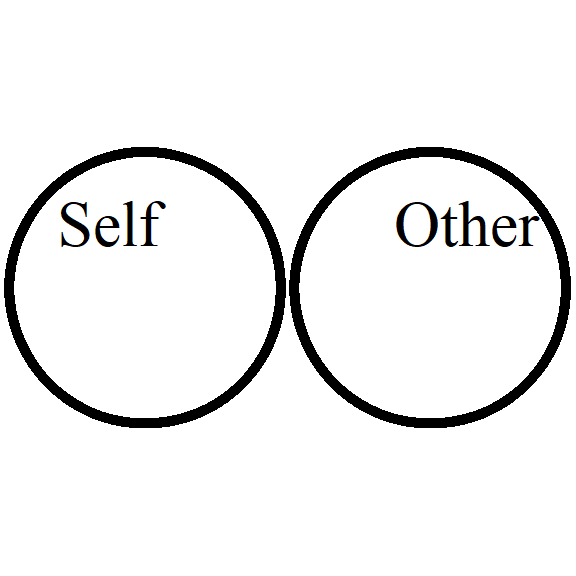### Score of 3.5 (25% overlap)

overlapCircle(3.5)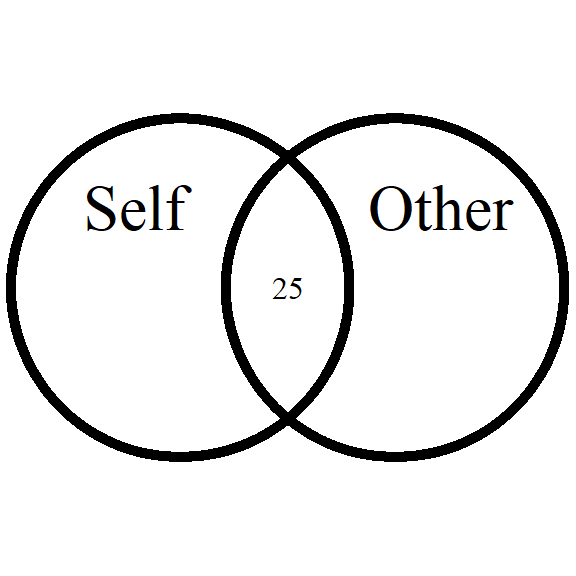### Score of 6.84 (81.8% overlap)

overlapCircle(6.84)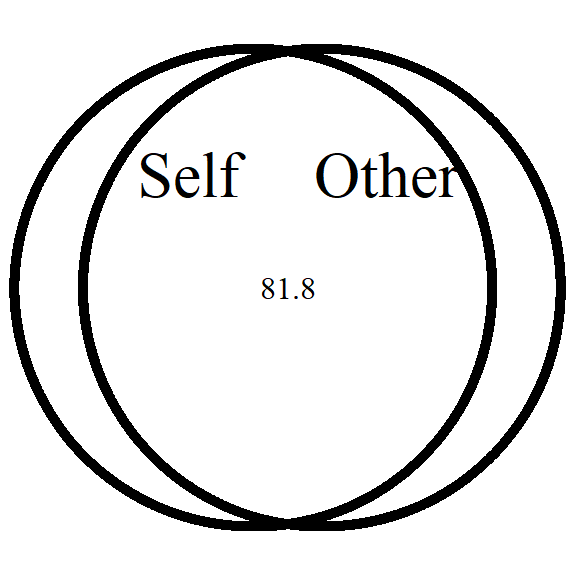# Changing labels

You can also change group labels with the categories argument, although ‘Self’ and ‘Other’ are the defaults.

overlapCircle(3.12, categories = c("Humans","Animals"))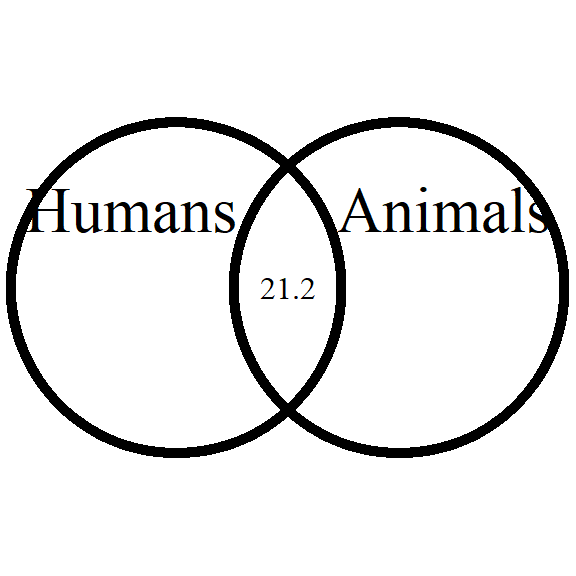# Saving to file (PDF or PNG)

First save the plot to an object:

plot <- overlapCircle(3.5)Then you can use the ggplot2 save command directly with the object name:

ggsave(plot, file="D:/R treasures/overlap.pdf", width=7, height=7, unit='in', dpi=300)
# Change the path to where you would like to save it.
# If you copy-paste your path name, remember to use "R" slashes ('/' rather than '\').
# Also remember to specify the .pdf extension of the file.

# Example figure

This allowed me for instance, for one study, to compare three of my groups side-by-side following an intervention: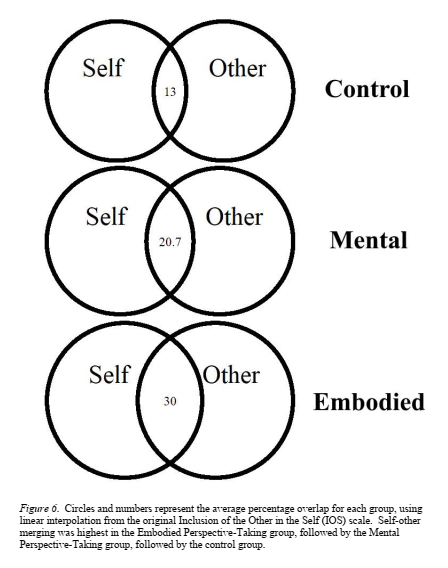### Concluding Statement

Make sure to check out this page again if you use the code after a time or if you encounter errors, as I periodically update or improve the code.

You can always edit the function to suit your purposes, or contact me for questions or requests to modify this function at https://remi-theriault.com/contact! Thanks for reading my guide! :) $$~$$

$$~$$

$$~$$

$$~$$

$$~$$

Updated 2021-01-02 (added: ggsave) $$~$$

$$~$$

$$~$$

$$~$$

$$~$$